# Canopy analysis in R using Forest Tools

## Introduction

The Forest Tools R package offers functions to analyze remotely sensed forest data. Currently, tools to detect dominant treetops and outline tree crowns have been implemented, both of which are applied to a rasterized canopy height model (CHM), which is generally derived from LiDAR or photogrammetric point clouds. A function to summarize the height and count of trees within user-defined geographical areas is also available.

The following vignette provides examples for using these functions.

## Installation

Check that R is up-to-date. This can be done automatically using the installr package. Alternatively, download the latest version directly from the Comprehensive R Archive Network (CRAN).

install.packages("installr")
library(installr)
updateR()

Download and install the Forest Tools package from CRAN using the install.packages function.

install.packages("ForestTools")

A sample canopy height model (CHM) is included in the Forest Tools package. It represents a small 1.5 hectare swath of forest in the Kootenay Mountains, British Columbia. The following examples use this sample, but if you would rather use your own data, it can be loaded into R using the raster function. A brief section on reading and writing geospatial data in R is included in this document. Otherwise, begin by loading the necessary libraries and the sample CHM using the library and data functions respectively.

# Attach the 'ForestTools' and 'raster' libraries
library(ForestTools)
library(raster)

# Load sample canopy height model
data("kootenayCHM")

View the CHM using the plot function. The cell values are equal to the canopy’s height above ground.

# Remove plot margins (optional)
par(mar = rep(0.5, 4))

# Plot CHM (extra optional arguments remove labels and tick marks from the plot)
plot(kootenayCHM, xlab = "", ylab = "", xaxt='n', yaxt = 'n')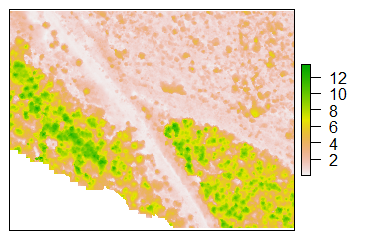## Detecting treetops

Dominant treetops can be detected using vwf. This function implements the variable window filter algorithm developed by Popescu and Wynne (2004). In short, a moving window scans the CHM, and if a given cell is found to be the highest within the window, it is tagged as a treetop. The size of the window itself changes depending on the height of the cell on which it is centered. This is to compensate for varying crown sizes, with tall trees having wide crowns and vice versa.

Therefore, the first step is to define the function that will define the dynamic window size. Essentially, this function should take a CHM cell value (i.e.: the height of the canopy above ground at that location) and return the radius of the search window. Here, we will define a simple linear equation, but any function with a single input and output will work.

lin <- function(x){x * 0.05 + 0.6}

We do not wish for the vwf to tag low-lying underbrush or other spurious treetops, and so we also set a minimum height of 2 m using the minHeight argument. Any cell with a lower value will not be tagged as a treetop.

ttops <- vwf(CHM = kootenayCHM, winFun = lin, minHeight = 2)

We can now plot these treetops on top of the CHM.

# Plot CHM
plot(kootenayCHM, xlab = "", ylab = "", xaxt='n', yaxt = 'n')

# Add dominant treetops to the plot
plot(ttops, col = "blue", pch = 20, cex = 0.5, add = TRUE)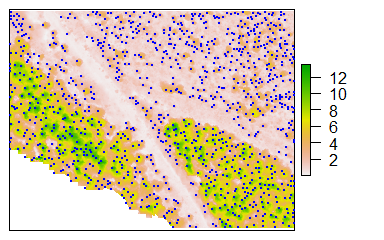The ttops object created by vwf in this example contains the spatial coordinates of each detected treetop, as well as two default attributes: height and winRadius. These correspond to the tree’s height above ground and the radius of the moving window where the tree was located. Note that winRadius is not necessarily equivalent to the tree’s crown radius.

# Get the mean treetop height
mean(ttops$height) ##  5.404217 ## Outlining tree crowns Canopy height models often represent continuous, dense forests, where tree crowns abut against each other. Outlining discrete crown shapes from this type of forest is often referred to as canopy segmentation, where each crown outline is represented by a segment. Once a set of treetops have been detected from a canopy height model, the mcws function can be used for this purpose. The mcws function implements the watershed algorithm from the imager library. Watershed algorithms are frequently used in topographical analysis to outline drainage basins. Given the morphological similarity between an inverted canopy and a terrain model, this same process can be used to outline tree crowns. However, a potential problem is the issue of oversegmentation, whereby branches, bumps and other spurious treetops are given their own segments. This source of error can be mitigated by using a variant of the algorithm known as marker-controlled segmentation (Beucher & Meyer, 1993), whereby the watershed algorithm is constrained by a set of markers–in this case, treetops. The mcws function also takes a minHeight argument, although this value should be lower than that which was assigned to vwf. For the latter, minHeight defines the lowest expected treetop, whereas for the former it should correspond to the height above ground of the fringes of the lowest trees. # Create crown map crowns <- mcws(treetops = ttops, CHM = kootenayCHM, minHeight = 1.5, verbose = FALSE) # Plot crowns plot(crowns, col = sample(rainbow(50), length(unique(crowns[])), replace = TRUE), legend = FALSE, xlab = "", ylab = "", xaxt='n', yaxt = 'n')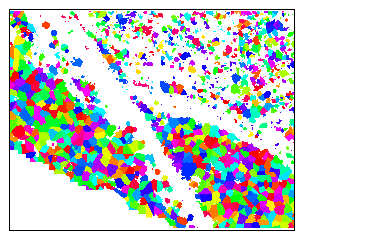By default, mcws returns a raster, where each crown is given a unique cell value. Depending on the intended purpose of the crown map, it may be preferable to store these outlines as polygons. Setting the format argument to “polygons” will convert the rasterized crown map to a set of polygons (a SpatialPolygonsDataFrame). As an added benefit, these polygons will inherit the attributes of the treetops from which they were generated, such as height. Furthermore, an extra attribute, crownArea, will be calculated for each polygon. It should be noted, however, that producing crown outlines as polygons requires significantly more processing time and disk space. # Create polygon crown map crownsPoly <- mcws(treetops = ttops, CHM = kootenayCHM, format = "polygons", minHeight = 1.5, verbose = FALSE) # Plot CHM plot(kootenayCHM, xlab = "", ylab = "", xaxt='n', yaxt = 'n') # Add crown outlines to the plot plot(crownsPoly, border = "blue", lwd = 0.5, add = TRUE)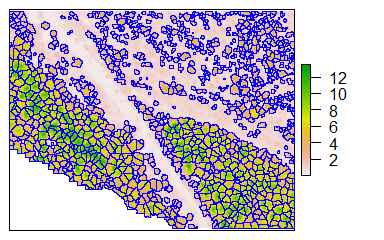Assuming that each crown has a roughly circular shape, we can use the crown’s area to compute its average circular diameter. # Compute average crown diameter crownsPoly[["crownDiameter"]] <- sqrt(crownsPoly[["crownArea"]]/ pi) * 2 # Mean crown diameter mean(crownsPoly$crownDiameter)
##  2.882985

## Spatial statistics

Managed forests are often divided into discrete spatial units. In British Columbia, for instance, these can range from cut blocks measuring a few hectares to timber supply areas, spanning several hundred square kilometers. The forest composition within these spatial units can be characterized through summarized statistics of tree attributes. For instance, a timber license holder may want a rough estimate of the number of dominant trees within a woodlot, while the standard deviation of tree height is of interest to anyone mapping heterogeneous old growth forest.

The sp_summarise function can be used to count trees within a set of spatial units, as well as compute statistics of the trees’ attributes. These spatial units can be in the form of spatial polygons, or can be generated in the form of a raster grid.

When no specific area is defined, sp_summarise will simply return the count of all inputted trees.

sp_summarise(ttops)
##           Value
## TreeCount  1077

Tree crowns can also be used as input. By defining the variables argument, sp_summarise will generate summarized statistics of the trees’ attributes. By default, the mean, median, standard deviation, minimum and maximum are computed, but custom functions can also be used.

sp_summarise(crownsPoly, variables = c("crownArea", "height"))
##                       Value
## TreeCount       1077.000000
## crownAreaMean      7.454271
## crownAreaMedian    6.250000
## crownAreaSD        5.398044
## crownAreaMin       0.250000
## crownAreaMax      53.000000
## heightMean         5.404217
## heightMedian       4.487972
## heightSD           2.969385
## heightMin          2.002042
## heightMax         13.491207

### Statistics by polygon

The Forest Tools package includes the boundaries of three cutting blocks that can be overlayed on kootenayCHM. Tree counts and height statistics can be summarized within these boundaries using the areas argument.

data("kootenayBlocks")

# Compute tree count and height statistics for cut blocks
blockStats <- sp_summarise(ttops, areas = kootenayBlocks, variables = "height")

# Plot CHM
plot(kootenayCHM, xlab = "", ylab = "", xaxt='n', yaxt = 'n')

# Add block outlines to the plot
plot(kootenayBlocks, add = TRUE, border =  "darkmagenta", lwd = 2)

# Add tree counts to the plot
library(rgeos)
text(gCentroid(kootenayBlocks, byid = TRUE), blockStats[["TreeCount"]], col = "darkmagenta", font = 2)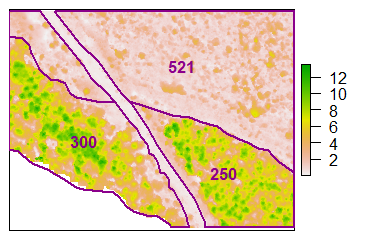# View height statistics
blockStats@data
##   BlockID Shape_Leng Shape_Area TreeCount heightMean heightMedian heightSD
## 0     101   304.3290   3706.389       300   7.538894     7.691572 2.587075
## 1    3308   508.6240   6712.607       521   3.111031     2.771317 1.003606
## 2     113   239.5202   2767.266       250   7.635933     8.180647 2.510343
##   heightMin heightMax
## 0  2.331073 13.491207
## 1  2.002042  7.125149
## 2  2.053920 12.583441

### Statistics by grid

Instead of defining polygonal areas, the sp_summarise function can also generate counts and stastics in raster format. In this case, the grid argument should be used instead of areas. If you have an existing raster with the extent, cell size and alignment that you would like to use, it can be input as the grid argument. Otherwise, simply entering a numeric value will generate a raster with that cell size.

# Compute tree count within a 10 m x 10 m cell grid
gridCount <- sp_summarise(ttops, grid = 10)

# Plot grid
plot(gridCount, col = heat.colors(255), xlab = "", ylab = "", xaxt='n', yaxt = 'n')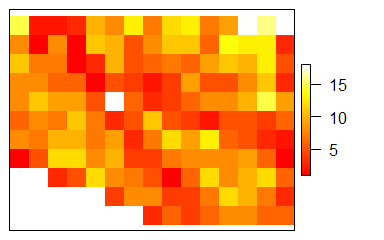If, in addition to tree count, tree attribute statistics are computed, the object returned by sp_summarise will be a RasterBrick, i.e.: a multi-layered raster.

# Compute tree height statistics within a 10 m x 10 m cell grid
gridStats <- sp_summarise(trees = ttops, grid = 10, variables = "height")

# View layer names
names(gridStats)
##  "TreeCount"    "heightMean"   "heightMedian" "heightSD"     "heightMin"
##  "heightMax"

Use the [[]] subsetting operator to extract a single layer.

# Plot mean tree height within 10 m x 10 m cell grid
plot(gridStats[["heightMean"]], col = heat.colors(255), xlab = "", ylab = "", xaxt='n', yaxt = 'n')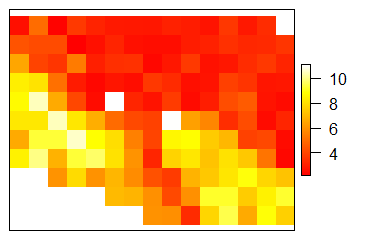### Using custom functions

By default, the statistics generated by sp_summarise for each attribute will be its mean, median, standard deviation, minimum and maximum. However, by using the statFuns argument, custom functions can be used instead.

Any custom function should observe the following conditions:

1. It should accept numeric vectors.
2. It should handle NA values.
3. It should have a single argument followed by an ellipsis (three dots). i.e.: function(x, ...)
4. It should return a single numeric value.

For instance, the following function will calculate the 98th quantile of a numeric vector.

quant98 <- function(x, ...) quantile(x, c(.98), na.rm = TRUE)

To have this function applied using sp_summarise, it must be put into a named list. Naming the functions in the list is needed for labeling the function’s outputs.

# Create list of functions
custFuns <- list(quant98, max)
names(custFuns) <- c("98thQuantile", "Max")

# Generate statistics for crown areas and tree heights
sp_summarise(crownsPoly, variables = c("crownArea", "height"), statFuns = custFuns)
##                            Value
## TreeCount             1077.00000
## crownArea98thQuantile   20.87000
## crownAreaMax            53.00000
## height98thQuantile      11.57175
## heightMax               13.49121

## Reading and writing geospatial data in R

### The raster and sp libraries

The Forest Tools package is built on the raster and sp libraries, which are automatically installed when ForestTools is downloaded. These libraries define a variety of classes and functions for working with raster and vector datasets in R.

It is recommended that any user performing geospatial analyses in R be familiar with both of these libraries.

### Geospatial classes used by Forest Tools

Data product Data type Object class
Canopy height model Single-layer raster RasterLayer
Treetops Points SpatialPointsDataFrame
Crown outlines Polygons RasterLayer, SpatialPolygonsDataFrame
Gridded statistics Multi-layer raster RasterLayer, RasterBrick

### Raster files

To load a raster file, such as a CHM, use the raster function from the raster library (both the function and the library have the same name). Simply provide a path to a valid raster file. Don’t forget to use either double backslashes \\ or forward slashes / in the file path.

library(raster)

# Load a canopy height model
inCHM <- raster("C:\\myFiles\\inputs\\testCHM.tif")

Once you have performed your analysis, use the writeRaster function to save any raster files you have produced. Setting an appropriate dataType is optional, but can save disk space.

# Write a crown map raster file
writeRaster(crowns, "C:\\myFiles\\outputs\\crowns.tif", dataType = "INT2U")

### Polygon and point files

There are many options for saving point and polygon files to disk. The rgdal library provides functions for reading and writing the most common vector formats. The following examples use ESRI Shapefiles.

Use the readOGR function to load a polygonal ESRI Shapefile. Instead of providing an entire file path, readOGR takes two separate arguments: the file’s directory, followed by the file name without an extension. The following would import a file named “C:\myFiles\blockBoundaries\block375.shp”.

library(rgdal)

blk375boundary <- readOGR("C:\\myFiles\\blockBoundaries", "block375")
Follow this same convention for saving a vector file to disk using writeOGR. A driver must also be specified.
# Save a set of dominant treetops
writeOGR(ttops, "C:\\myFiles\\outputs", "treetops", driver = "ESRI Shapefile")